# Create a Pandas DataFrame from Lists

Python is a great language for doing data analysis, primarily because of the fantastic ecosystem of data-centric python packages. Pandas is one of those packages and makes importing and analyzing data much easier.

Creating Pandas Dataframe can be achieved in multiple ways. Let’s see how can we create a Pandas DataFrame from Lists.

Code #1: Basic example

 `# import pandas as pd ` `import` `pandas as pd ` ` `  `# list of strings ` `lst ``=` `[``'Geeks'``, ``'For'``, ``'Geeks'``, ``'is'``,  ` `            ``'portal'``, ``'for'``, ``'Geeks'``] ` ` `  `# Calling DataFrame constructor on list ` `df ``=` `pd.DataFrame(lst) ` `df `

Output: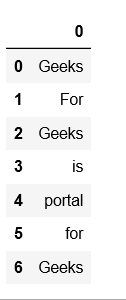Code #2: Dataframe using list with index and column names

 `# import pandas as pd ` `import` `pandas as pd ` ` `  `# list of strings ` `lst ``=` `[``'Geeks'``, ``'For'``, ``'Geeks'``, ``'is'``, ``'portal'``, ``'for'``, ``'Geeks'``] ` ` `  `# Calling DataFrame constructor on list ` `# with indices and columns specified ` `df ``=` `pd.DataFrame(lst, index ``=``[``'a'``, ``'b'``, ``'c'``, ``'d'``, ``'e'``, ``'f'``, ``'g'``], ` `                                              ``columns ``=``[``'Names'``]) ` `df `

Output: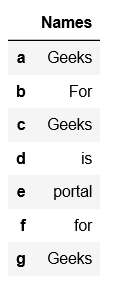Code #3: Using zip() for zipping two lists

 `# import pandas as pd ` `import` `pandas as pd ` ` `  `# list of strings ` `lst ``=` `[``'Geeks'``, ``'For'``, ``'Geeks'``, ``'is'``, ``'portal'``, ``'for'``, ``'Geeks'``] ` ` `  `# list of int ` `lst2 ``=` `[``11``, ``22``, ``33``, ``44``, ``55``, ``66``, ``77``] ` ` `  `# Calling DataFrame constructor after zipping ` `# both lists, with columns specified ` `df ``=` `pd.DataFrame(``list``(``zip``(lst, lst2)), ` `               ``columns ``=``[``'Name'``, ``'val'``]) ` `df `

Output: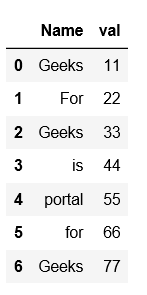Code #4: Creating DataFrame using multi-dimensional list

 `# import pandas as pd ` `import` `pandas as pd  ` `   `  `# List1  ` `lst ``=` `[[``'tom'``, ``25``], [``'krish'``, ``30``], ` `       ``[``'nick'``, ``26``], [``'juli'``, ``22``]] ` `   `  `df ``=` `pd.DataFrame(lst, columns ``=``[``'Name'``, ``'Age'``]) ` `df `

Output: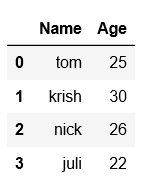Code #5: Using multi-dimensional list with column name and dtype specified.

 `# import pandas as pd ` `import` `pandas as pd  ` `   `  `# List1  ` `lst ``=` `[[``'tom'``, ``'reacher'``, ``25``], [``'krish'``, ``'pete'``, ``30``], ` `       ``[``'nick'``, ``'wilson'``, ``26``], [``'juli'``, ``'williams'``, ``22``]] ` `   `  `df ``=` `pd.DataFrame(lst, columns ``=``[``'FName'``, ``'LName'``, ``'Age'``], dtype ``=` `float``) ` `df `

Output: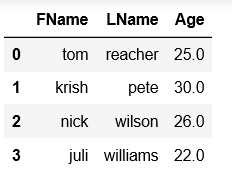Code #6: Using lists in dictionary to create dataframe

 `# importing pandas as pd  ` `import` `pandas as pd  ` ` `  `# list of name, degree, score ` `nme ``=` `[``"aparna"``, ``"pankaj"``, ``"sudhir"``, ``"Geeku"``] ` `deg ``=` `[``"MBA"``, ``"BCA"``, ``"M.Tech"``, ``"MBA"``] ` `scr ``=` `[``90``, ``40``, ``80``, ``98``] ` ` `  `# dictionary of lists  ` `dict` `=` `{``'name'``: nme, ``'degree'``: deg, ``'score'``: scr}  ` `   `  `df ``=` `pd.DataFrame(``dict``) ` `   `  `df  `

Output:My Personal Notes arrow_drop_upCheck out this Author's contributed articles.

If you like GeeksforGeeks and would like to contribute, you can also write an article using contribute.geeksforgeeks.org or mail your article to contribute@geeksforgeeks.org. See your article appearing on the GeeksforGeeks main page and help other Geeks.

Please Improve this article if you find anything incorrect by clicking on the "Improve Article" button below.

Article Tags :

3

Please write to us at contribute@geeksforgeeks.org to report any issue with the above content.